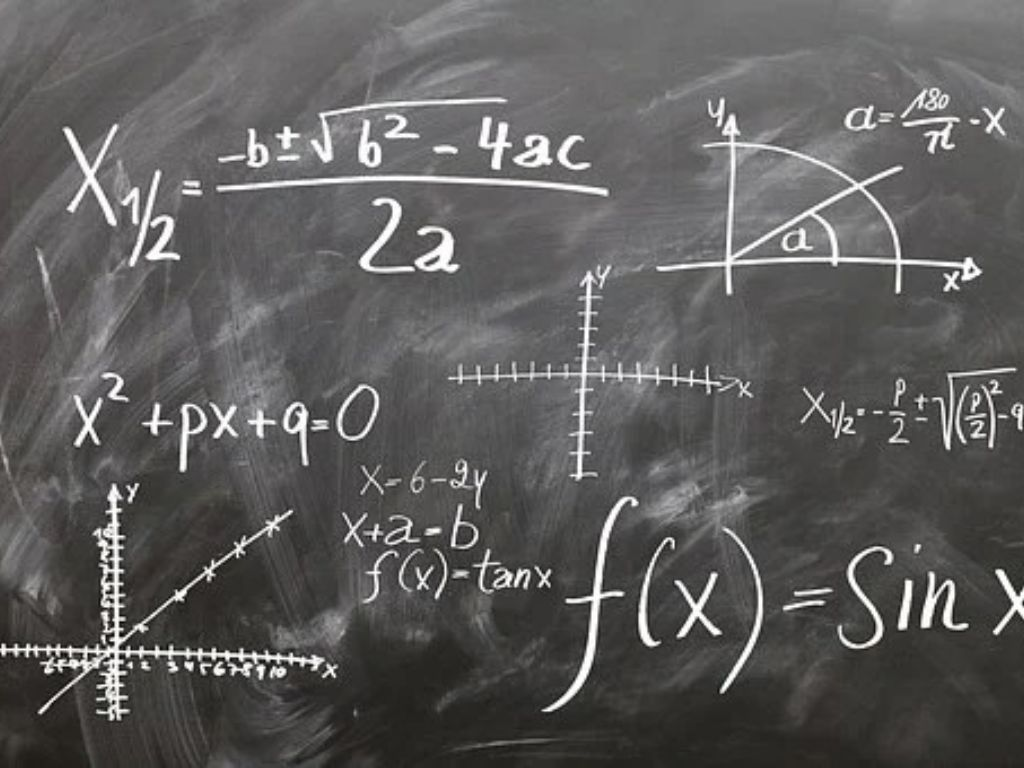## Quadratic Formula (Shridharacharya Sutra) Class 10th

Introduction Quadratic Formula (Shridharacharya Sutra) – The quadratic formula is given by the great Indian mathematician Shridharacharya and is also known as Shridharacharya Sutra. This formula is used to solve the quadratic equation. x = –b ± √(b2 – 4ac)/2a Where, x = variable a, b, c = Coefficients of quadratic equation Derivation of Formula …